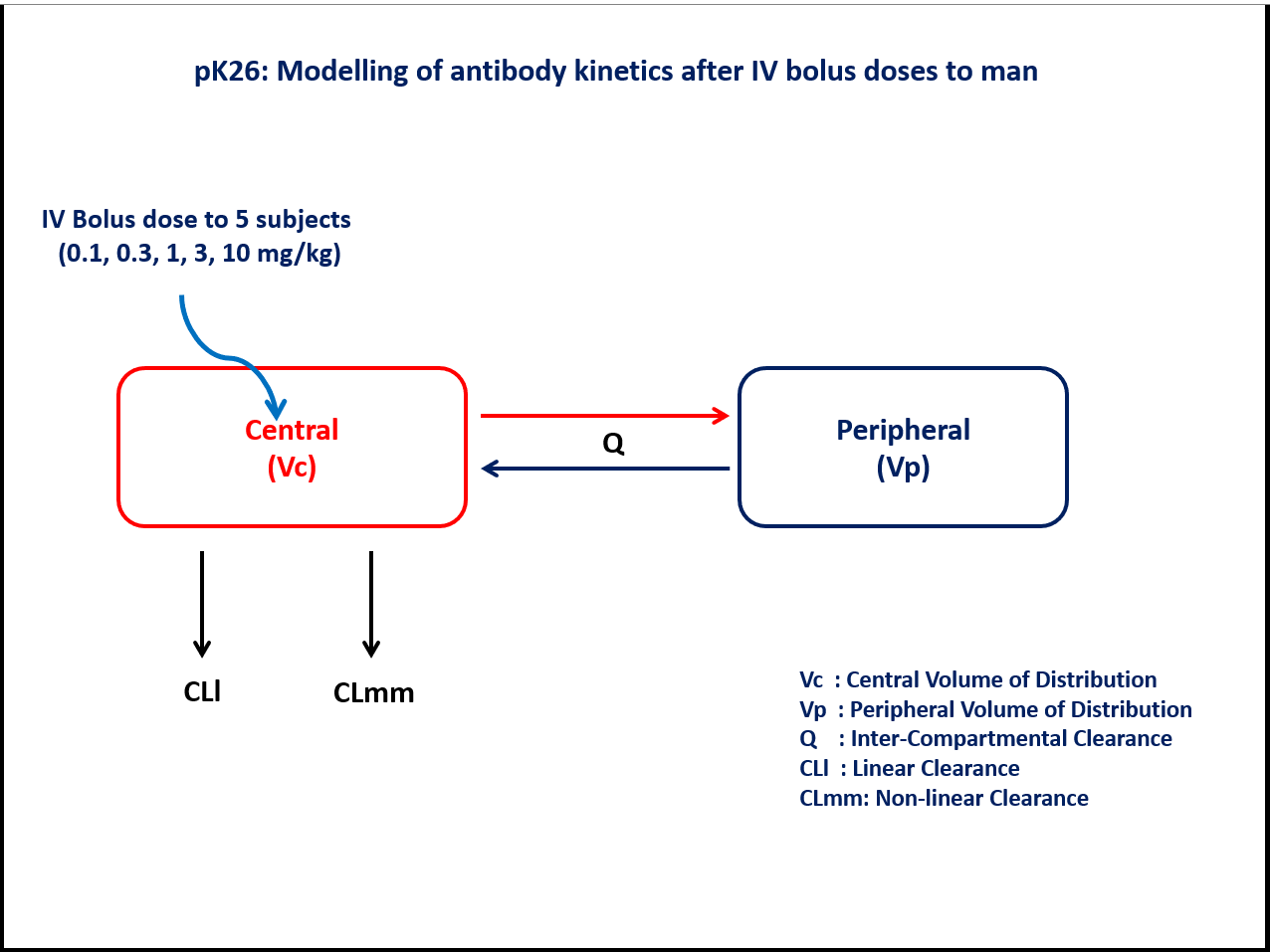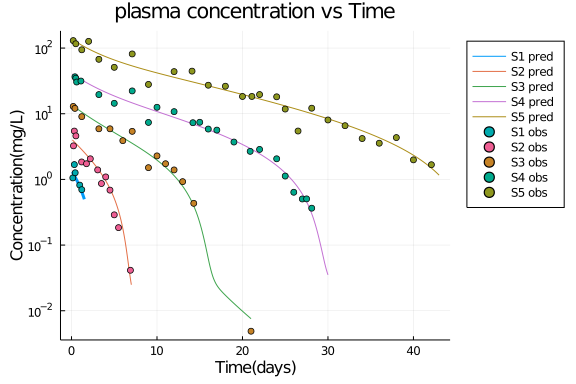# Exercise PK26 - Modelling of antibody kinetics after IV doses to man

### Background

Following info:

• Structural model - Two compartment model with parallel linear and Non-linear elimination

• Route of administration - IV bolus (Single dose)

• Dosage Regimen - 0.1mg/kg, 0.3mg/kg, 1mg/kg, 3mg/kg, and 10 mg/kg

• Number of Subjects - 5### Learning Outcomes

To understand the antibody kinetics with linear and nonlinear elimination after IV bolus dose in man.

### Objectives

• To build a two compartment model with parallel linear and non-linear elimination to understand the antibody kinetics.

• To simulate dataset for 5 subjects after single dose IV bolus administration

### Libraries

Call the "necessary" libraries to get started.

using Pumas
using Plots
using CSV
using StatsPlots
using DataFrames
using Random


### Model

Two compartment model with parallel linear and Non- linear elimination

PK26            = @model begin
@param begin
tvvmax      ∈ RealDomain(lower=0)
tvkm        ∈ RealDomain(lower=0)
tvvp        ∈ RealDomain(lower=0)
tvvc        ∈ RealDomain(lower=0)
tvq         ∈ RealDomain(lower=0)
tvcll       ∈ RealDomain(lower=0)
Ω           ∈ PDiagDomain(6)
σ           ∈ RealDomain(lower=0)
end

@random begin
η           ~ MvNormal(Ω)
end

@pre begin
Vmax        = tvvmax*exp(η)
Km          = tvkm*exp(η)
Vp          = tvvp*exp(η)
Vc          = tvvc*exp(η)
Q           = tvq*exp(η)
CLl         = tvcll*exp(η) # Linear clearance
# CLmm       = Vmax/(Km+C)     Non-linear clearance
end

@dynamics begin
Central'    = -(Vmax/(Km+(Central/Vc)))*(Central/Vc) - CLl*(Central/Vc)-(Q/Vc)*Central +(Q/Vp)*Peripheral
Peripheral' = (Q/Vc)*Central -(Q/Vp)*Peripheral
end

@derived begin
cp          = @. Central/Vc
dv          ~ @. Normal(cp, sqrt(cp^2*σ))
end
end

PumasModel
Parameters: tvvmax, tvkm, tvvp, tvvc, tvq, tvcll, Ω, σ
Random effects: η
Covariates:
Dynamical variables: Central, Peripheral
Derived: cp, dv
Observed: cp, dv


### Parameters

The parameters are as given below. tv represents the typical value for parameters.

• Vmax - Maximum rate of Metabolism(mg/hr/kg)

• Km - Michaelis constant (mg/L/kg)

• Vp - Volume of Peripheral Compartment(L/kg)

• Vc - Volume of Central Compartment(L/kg)

• Q - Inter-compartmental Clearance(L/hr/kg)

• CLl - Linear Clearance(L/hr/kg)

• Ω - Between Subject Variability

• σ - Residual error

param = ( tvvmax  = 0.0338,
tvkm    = 0.0760,
tvvp    = 0.0293,
tvvc    = 0.0729,
tvq     = 0.0070,
tvcll   = 0.0069,
Ω       = Diagonal([0.0,0.0,0.0,0.0,0.0,0.0]),
σ       = 0.04)

(tvvmax = 0.0338, tvkm = 0.076, tvvp = 0.0293, tvvc = 0.0729, tvq = 0.007,
tvcll = 0.0069, Ω = [0.0 0.0 … 0.0 0.0; 0.0 0.0 … 0.0 0.0; … ; 0.0 0.0 … 0.
0 0.0; 0.0 0.0 … 0.0 0.0], σ = 0.04)


### Dosage Regimen

5 subjects received an IV bolus dose of 0.1, 0.3, 1, 3 and 10 mg/kg respectively at time=0

DR1 = DosageRegimen(0.1, time=0)
DR2 = DosageRegimen(0.3, time=0)
DR3 = DosageRegimen(1, time=0)
DR4 = DosageRegimen(3, time=0)
DR5 = DosageRegimen(10, time=0)
s1  = Subject(id=1, events=DR1)
s2  = Subject(id=2, events=DR2)
s3  = Subject(id=3, events=DR3)
s4  = Subject(id=4, events=DR4)
s5  = Subject(id=5, events=DR5)
pop = Population([s1,s2,s3,s4,s5])

Population
Subjects: 5
Covariates:


### Simulation

To simulate plasma concentration data for 5 subjects with specific obstimes.

Random.seed!(123)
sim1 = simobs(PK26,s1,param,obstimes =   0.1:0.01: 1.5)
Random.seed!(123)
sim2 = simobs(PK26,s2,param,obstimes =   0.1:0.01:7)
Random.seed!(123)
sim3 = simobs(PK26,s3,param,obstimes =   0.1:0.1:21)
Random.seed!(123)
sim4 = simobs(PK26,s4,param,obstimes =   0.1:0.1:30)
Random.seed!(123)
sim5 = simobs(PK26,s5,param,obstimes =   0.1: 0.1:43)
sim  = [sim1,sim2,sim3,sim4,sim5]


### Dataframe and Plots

df1    = DataFrame(sim1)
df1_dv = filter(x -> x.time in [0.07, 0.15, 0.33, 0.43, 0.96, 1.2],df1)
df2    = DataFrame(sim2)
df2_dv = filter(x -> x.time in [0.23, 0.33, 0.51, 1.18, 1.75, 2.2, 3.06, 3.5, 4, 4.5, 5, 5.5, 6.89],df2)
df3    = DataFrame(sim3)
df3_dv = filter(x -> x.time in [0.2, 0.4, 1.2, 3.2, 4.5, 6, 7.1, 9, 10, 11, 12, 13, 14.3,21],df3)
df4    = DataFrame(sim4)
df4_dv = filter(x -> x.time in [0.4, 0.5, 0.6, 1.1, 3.2, 5, 7.1, 9, 10, 12, 14.2, 15, 16, 17, 19, 20.9, 22, 24, 25, 26, 27, 27.5, 28.1],df4)
df5    = DataFrame(sim5)
df5_dv = filter(x -> x.time in [0.2, 0.5, 1.2, 2, 3.2, 5, 7.1, 9, 12, 14.1, 16, 18, 20, 21.1, 22, 24, 25, 26.5, 28.1, 30, 32, 34, 36, 38, 40, 42.1],df5)

DF  = [df1_dv,df2_dv,df3_dv,df4_dv,df5_dv]
DF = vcat(df1_dv,df2_dv,df3_dv,df4_dv,df5_dv)

@df df1 plot(:time, :cp,
title = "plasma concentration vs Time", label ="S1 pred",
yaxis =:log, xlabel = "Time(days)", ylabel = "Concentration(mg/L)",
linewidth =3,legend = :outertopright)
@df df2 plot!(:time, :cp,label= "S2 pred" )
@df df3 plot!(:time, :cp,label= "S3 pred")
@df df4 plot!(:time, :cp,label= "S4 pred")
@df df5 plot!(:time, :cp,label= "S5 pred")
@df df1_dv scatter!(:time, :dv, label= "S1 obs")
@df df2_dv scatter!(:time, :dv, label= "S2 obs")
@df df3_dv scatter!(:time, :dv, label= "S3 obs")
@df df4_dv scatter!(:time, :dv, label= "S4 obs")
@df df5_dv scatter!(:time, :dv, label= "S5 obs")